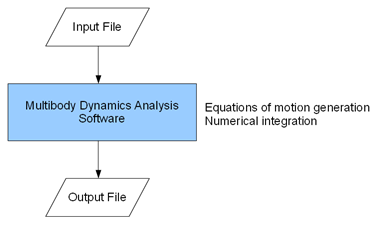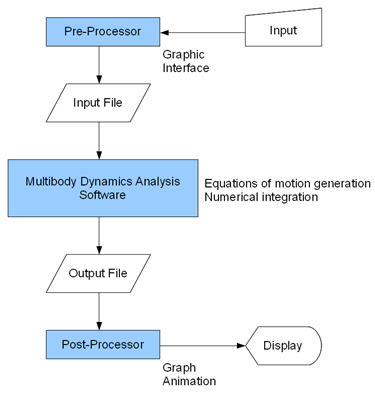Home > Resources > MBDyn Tutorial > 2. Multibody dynamics analysis basics
MBDyn Tutorial

## 2. Multibody dynamics analysis basics

Before getting into specifics of MBDyn, we briefly review in general terms what multibody dynamics analysis is and what general-purpose multibody dynamics analysis software is like.

### What is multibody dynamics analysis?

In multibody dynamics analysis, we are concerned with dynamics of mutually-interconnected multiple rigid bodies. The purpose of this analysis is to find how those bodies move as a system and what forces are generated in the process. This is typically done numerically using computers.

As an example, consider a mechanism shown in Figure 1.Figure 1: Double rigid pendulum

This is a mechanism called "double rigid pendulum," where two rigid bodies mutually connected with a hinge are hung from a ceiling with another hinge. If the end of the pendulum is raised and released, the pendulum starts to oscillate. How the position and the velocity of the pendulum change with time and what forces act on the two hinges when the pendulum is in motion can be obtained by multibody dynamics analysis.
( See animation >> )

In general, in order to compute the motion of bodies it is required first to formulate the equations of motion and second to integrate them. In multibody dynamics analysis, the equations of motion are formulated in some deterministic manner from information of each rigid body (mass, center of mass, inertia tensor) and interaction conditions between bodies (forces, constraints). The equations of motion are then integrated and solved numerically by a program called ODE (or DAE) solver. (ODE = ordinary differential equation, DAE = differential algebraic equation.)

### What is general-purpose multibody dynamics analysis software?

General-purpose multibody dynamics analysis software such as MBDyn enables us to carry out multibody dynamics analysis for an arbitrary multibody system. Thus, if a user inputs information of rigid bodies and interaction conditions between bodies for an arbitrary multibody system, this software automatically generates the equations of motion, numerically integrates them, and returns the motion of the system to the user. So, with this software the user is able to study motions of a complex multibody system easily even if he is not an expert of dynamics.

### Input and output of multibody dynamics analysis

Typical input and output data for multibody dynamics analysis are as follows.

Input data:

• Rigid bodies (mass, center of mass, inertia tensor)
• Constraints (joints, prescribed motions, etc.)
• Forces (springs, dampers, frictions, contact forces, gravity, external forces, etc.)
• Initial conditions (initial position, initial velocity, initial orientation, initial angular velocity)
• Integration parameters (choice of solver, step size, tolerance, simulation time, etc.)

Output data:

• Motions of rigid bodies (position, velocity, orientation, angular velocity)
• Joint reaction forces
• Other forces

Input data are coded in an input file. Multibody dynamics analysis software reads the input file and executes a multibody dynamics analysis. The output data, motions and forces, are then written in some output files. (See Figure 2.)Figure 2: Input and output of general-purpose multibody dynamics analysis software

### Pre-processor and post-processor

Many of commercial multibody dynamics analysis software packages include programs called pre-processor and post-processor. A pre-processor is a program whose function is mainly to allow the user to create an input file through graphic interface manipulations. A post-processor is a program whose function is mainly to make graphs and animations from output data. Both programs are to support the user to conduct multibody dynamics analysis more conveniently. (See Figure 3.)Figure 3: Pre-processor and post-processor

MBDyn, however, does not have its own pre-processor and post-processor at present. Without a pre-processor, the user has to write input files directly in a specific format. This may require the user some practice. But once the user masters writing the input file, there is an advantage that he is able to control the input much more freely than possibly through a pre-processor. Some techniques to write effective input files are also discussed in this tutorial.

Without a post-processor, the user has to use external programs to make graphs and animations. To make graphs, numerical analysis software with basic plotting functions such as MATLAB, Scilab, or Octave can be used. To make animations, EasyAnim, which is maintained by the MBDyn project team, may be used. It has also been proposed to use software like Blender, free 3D graphics software, for animation. As an alternative, numerical analysis software such as MATLAB often has sophisticated graphics functionalities and may also be used to make animations. In fact, most animations in this tutorial including the one of the double rigid pendulum above are made using MATLAB. Some post-processing techniques are presented in the later chapters.